# CONSTRUCTAL TREE-SHAPED DESIGNS FOR SELF-COOLING

CONSTRUCTAL TREE-SHAPED DESIGNS FOR SELF-COOLING

O. YenigünE. Çetkin

Izmir Institute of Technology, Department of Mechanical Engineering, Urla, Izmir 35430, Turkey

Corresponding Author Email:
onuryenigun@iyte.edu.tr
Page:
S173-S178
|
DOI:
https://dx.doi.org/10.18280/ijht.34S123
|
Accepted:
|
Published:
31 January 2016
| Citation

OPEN ACCESS

Abstract:

In this paper, we show how a plate which is subjected to a heating load can be kept under an allowable temperature. Vascular channels in which coolant fluid flows have been embedded in the plate. Two types of vascular channel designs were compared: radial and tree-shaped. The effects of channel design on the thermal performance for different volume fractions (the fluid volume over the solid volume) are documented. Changing the design from radial to tree-shaped designs decreases the order of pressure drop. Hence increase in the order of the convection coefficient is achieved. However, tree-shaped designs do not bath the entire domain. Therefore, we have inserted additional branches at the uncooled regions. Then, we have compared the peak temperatures of radial, traditional tree-shaped and improved tree-shaped designs. The effect of design on the maximum temperature shows that there should be an optimum design for a distinct set of boundary conditions, and this design should be varied as the boundary conditions change. This result is in accord with the constructal law, i.e. the shape should be varied in order to minimize resistances to the flows.

Keywords:

Constructal law, Self-cooling, Vascular, Radial, Tree-shaped.

1. Introduction

Advanced technologies require great volumetric cooling capabilities due to the trend of miniaturization. The heat transfer surface area cannot be increased in miniature designs. Therefore, the current literature focuses on heat transfer enhancement with nanofluids and phase change [1-4]. These methods are essential in order to increase the heat transfer. However, heat load can be of two kinds: deterministic and random . The heating rate and the surface on which the heat flux is applied is known in deterministic kind of heating loads such as electronic circuits. In random type loads, neither heating rate nor the applied surface are known such as thermal runaway in an accumulator. Therefore, future technologies require cooling systems capable of cooling the entire structure under deterministic and random loads. This requirement can be satisfied with self-cooling materials [5-12].

Materials with smart features first suggested by White et. al. in 2001 . They mimicked the self-healing mechanism of animals, i.e. clot appearance at the wound in order to seal it. However, in their autonomic healing concept healing agents were placed in spheres which can be used only once. Later, Hamilton et. al. discussed that vascularizing the structure enables it to heal itself countless time similar to the circulatory systems of warm blooded animals . Bejan et. al. showed that vascularized structures can also be used to cool a domain on which heat load applied. . Wang et. al. uncovered how the mechanical strength of a vascular domain varies with changing the channel design and volume fraction . Later, literature showed how the mechanical strength and cooling performance is affected by the volume fraction and by the shape of the channel configurations . Cetkin et. al. uncovered that vascularization provides required cooling both deterministic and random cooling requirements . Then, the current literature also shows how vascularized structures increases the mechanical strength of a heated domain with applied mechanical load and thermal expansion [17-18].

This paper discusses the novel hybrid and tree-shaped structures in order to gain self-cooling capability to solid structures. Embedded cooling channels inside the solid domain decreases the resistances to the heat flow on the plate surface. Therefore, even the heat load is local and random, the peak temperature is kept lower than the structures without embedded vascular channels. Here, we discuss that the vascularized structures are not a replacement for phase change and nanofluid cooling methods but a supplement for these methods, i.e., explicitly where the heating load is random. In summary, vascular self-cooling materials promise to create a heat exchanger from the solid domain. Therefore, unpredictable and random heating loads will not damage the structure.

2. Model

Consider a plate of size 120x120mm with embedded vascularized cooling channels which is subjected to constant heat flux of 5000 W/m2 from one of its surface boundary, as shown in Fig. 1. Coolant fluid flows along the embedded cooling channels. The fluid flow is driven by the pressure difference between the inlet and outlet boundaries of the cooling channels. The volume of the vascular channels is fixed, so is the volume of the solid material. Heat transfer symmetry boundary condition $(\partial T / \partial n=0)$ is applied for the outer boundary surfaces except the heated surface. The symmetry boundary condition is selected because the plate is an elemental domain of a greater system, which consists of N numbers of elemental domains as shown in Fig. 1.

## 1.png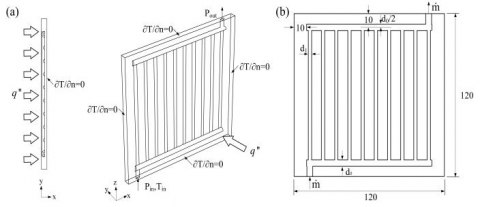Figure 1. The plate with embedded semi-circular radial cooling channels: (a) boundary conditions with perspective of the geometry, (b) dimensions of the geometry

The coolant fluid is water and its thermo-physical properties as a function of temperature are given in Table 1. In addition, the fluid flow is steady. With these are in mind, the conservation of mass and momentum equations can be written as

$\frac{\partial u}{\partial x}+\frac{\partial v}{\partial y}+\frac{\partial w}{\partial z}=0$(1)

$u \frac{\partial u}{\partial x}+v \frac{\partial u}{\partial y}+w \frac{\partial u}{\partial z}=-\frac{1}{\rho} \frac{\partial P}{\partial x}+\nu \nabla^{2} u$(2)

$u \frac{\partial v}{\partial x}+v \frac{\partial v}{\partial y}+w \frac{\partial v}{\partial z}=-\frac{1}{\rho} \frac{\partial P}{\partial y}+\nu \nabla^{2} v$(3)

$u \frac{\partial w}{\partial x}+v \frac{\partial w}{\partial y}+w \frac{\partial w}{\partial z}=-\frac{1}{\rho} \frac{\partial P}{\partial z}+\nu \nabla^{2} w$(4)

where $\nabla^{2}=\partial^{2} / \partial \mathrm{x}^{2}+\partial^{2} / \partial y^{2}+\partial^{2} / \partial z^{2}$, x, y and z are spatial coordinates, and the velocity components that correspond to these coordinates are u, v and w respectively. P, ρ and ν are the pressure, density and kinematic viscosity of the fluid.

The temperature distribution inside the fluid domain is calculated by solving the energy equation]

$\rho c_{p}\left(u \frac{\partial T}{\partial x}+v \frac{\partial T}{\partial y}+w \frac{\partial T}{\partial z}\right)=\frac{\partial}{\partial x}\left(\mathrm{k}_{f} \frac{\partial T}{\partial x}\right)+\frac{\partial}{\partial y}\left(\mathrm{k}_{f} \frac{\partial T}{\partial y}\right)+\frac{\partial}{\partial z}\left(\mathrm{k}_{f} \frac{\partial T}{\partial z}\right)$(5)

where T is the temperature, $c_{p}$ and $k_{f}$ are the specific heat at constant pressure and the thermal conductivity of the coolant fluid as given in Table 1. The heat continuity in between the solid and fluid domains require

$\left.k_{f} \frac{\partial T}{\partial n}\right|_{f}=\left.k_{s} \frac{\partial T}{\partial n}\right|_{s}$(6)

where $k_{s}$ is the thermal conductivity of the solid, n is the vector normal to the fluid-solid interface.

Table 1. Thermo-physical properties at atmospheric pressure 

## table_1.png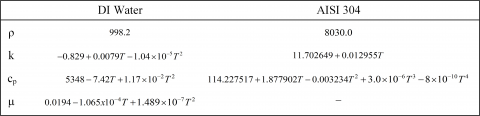3. Numerical Method

Consider the square slab of Figure 1a which has semi-circular vascularized channels embedded inside of it. The vascularized channels are placed in radial form with the total channel volume of $4 \times 10^{-6} m^{3}$. There are one distributing and one collecting channels with the radius of $r_{1}=2.337 \times 10^{-3} \mathrm{m}$, and there are ten daughter channels with the radius of $r_{2}=1.2945 \times 10^{-3} \mathrm{m}$. The surface, which the heating load of $q^{\prime \prime}=5000 \mathrm{W} / \mathrm{m}^{2}$ applied on, is shown in Fig. 1a. The coolant fluid enters to the main distributing channel with Pin inlet pressure which varies from 30Pa to 3kPa. The outer surfaces of the plate are symmetry boundaries, i.e., $(\partial T / \partial n=0)$, except the boundary, which the heating load is applied on.

Conservation of mass, momentum and energy equations were solved by using a commercial finite element software. Mesh elements are non-uniform in the domain, and boundary layer meshes are used in order to uncover the effect of gradient changes near the boundaries. The mesh size was changed until the criteria of $\left|\left(T_{\text {peak}}^{m}-T_{\text {peak}}^{m+1}\right) / T_{\text {peak}}^{m}\right|<5 \times 10^{-3}$ and $\left|\left(T_{p e a k}^{m}-T_{p e a k}^{m+1}\right) / T_{p e a k}^{m}\right|<5 \times 10^{-3}$ are satisfied. Relative errors corresponding to the mesh size can be seen in Table 2. Table 2 shows that the solution becomes mesh independent with 261248 number of mesh elements.

Table 2. Relative errors corresponding to the number of mesh elements

## table_2.png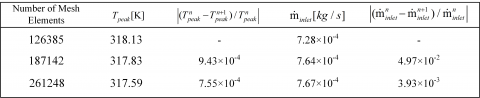The current numerical study is validated by using numerical and experimental data of Ref. . Figure 2 shows the current study agrees well with the results of Ref. . The mass flow rate error value is maximum 9% and 3% for numerical and experimental studies of Ref. , respectively. Therefore, we conclude that our simulation results are mesh independent and accurate in comparison with current the literature.

## 2.pngFigure 2. Validation of the current numerical method with the results of Ref. 

Consider the radial cooling channel design in Figure 1 with circular channels instead of semi-circular channels. The volume of the cooling channels and the solid domain are fixed, and they are the same as in section 3. In addition, ratio of r1 over r2 is also fixed. Figure 3a shows that the resistances to the fluid flow decrease by changing only the cross-section of the channels from semi-circular to circular. The result of Figure 3a was expected because the hydraulic diameter of semi-circular channels is smaller than in the circular channels for the same volume fraction. In addition, Figure 3b shows how the peak temperature varies as pressure drop changes for circular and semi-circular channels. Figure 3b shows that the peak temperature decreases as the cross-section is changed from semi-circular to circular channels when the fluid and solid volumes are fixed until pressure drop increases from 30 Pa to 1500 Pa. This design effect is more visible when pressure drop is smaller than 1000 Pa. Until this limit, the drop in the flow resistances provides smaller peak temperatures in circular cross-section channels than in semi-circular ones. However, if the pressure drop becomes greater than 1000 Pa, then this effect diminishes as enough coolant is received by the channels for cooling. As pressure drop is kept increased from 1500 Pa to 3000 Pa, semi-circular channels provide slightly smaller peak temperature (0.1%) in comparison with the circular channel configurations. The reason of this is that the radius of semi-circular channels are greater than in circular channels and they have the same point as origin. Therefore, the conductive resistances in circular channel configurations are greater than in the semi-circular channel configurations. The effect of this difference in conductive resistances becomes visible as convective resistances decrease greatly and their order becomes similar to the conductive resistances, i.e. convective heat transfer coefficient increases due to the increase in pressure drop.

Next, the volume fraction (φ) of the radial design with circular channels is changed from %6.5 to %5 and %8 in order to uncover the effects of φ on cooling performance. While changing the volume fraction, only the diameters of the cooling channels are varied. Increasing the volume fraction decreases the flow resistances due to the increase in the channel diameters. Figure 4a shows that how the peak temperature varies when pressure drop varies between 30 Pa to 350 Pa for the different volume fractions; φ=0.05, 0.065 and 0.08. The peak temperature decreases as the volume fraction increases, Fig. 4a. The reason for this decrease is that the resistances to the fluid flow decreases as channel diameters increase, and therefore the fluid gains greater access to the entire domain (i.e., convective and conductive resistances decrease).

## 3a.pngFigure 3. (a) Mass flow rate and (b) peak temperature with respect to the pressure drop for radial designs: semi-circular and circular channels

## 3b.png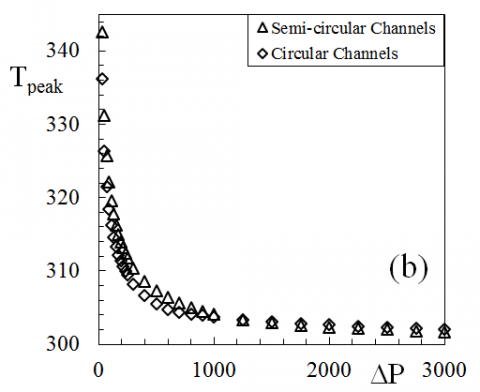Figure 4. Peak temperature variation with pressure drop (a) for radial design with volume fractions of φ=0.05, 0.065 and 0.08, (b) for radial design with 6 and 10 number of channels when φ=0.05

Next, consider the circular radial design with six daughter channels instead of ten. Since decreasing the number of channels increase the channel diameters (i.e., volume fraction is fixed), φ is decreased from %6.5 to %5 in order to prevent the channel diameters to become greater than the thickness of the solid plate. Figure 4b shows that the comparison of radial designs with six and ten daughter channels where φ=0.05. Figure 4b illustrates that the flow resistances of radial design with six daughter channels are smaller than the design with ten daughter channels as ΔP varies from 30Pa to 350Pa. Figure 4b shows that peak temperatures are smaller in the design with six channels than with ten channels. This means that for this specific volume fraction, the conductive resistances due to the spacing between channels are small enough; therefore, their effect is negligible.

5. Tree-Shaped Design

Next, consider a tree-shaped design with three levels of embedded vascular cooling channels as discussed in Ref. , i.e., four cooling channels of diameter d1 are connected to eight channels of diameter d2 and they are connected to sixteen daughter channels of diameter d3 as shown in Fig. 5. The tree-shaped channels are connected to one distributing and one collecting channels of diameter d0. The coolant fluid enters from the distributing channel of diameter d0, and then it is distributed to the vascular channels of diameter d1, d2 and d3, respectively or vice versa. The diameter ratios at the junctions are determined according to the Hess-Murray Rule 

$d_{i} / d_{i+1}=n_{u}^{1 / 3}$(7)

where nu is the number of daughter channels that are connected to the mother channel at the each junction.

## 5.png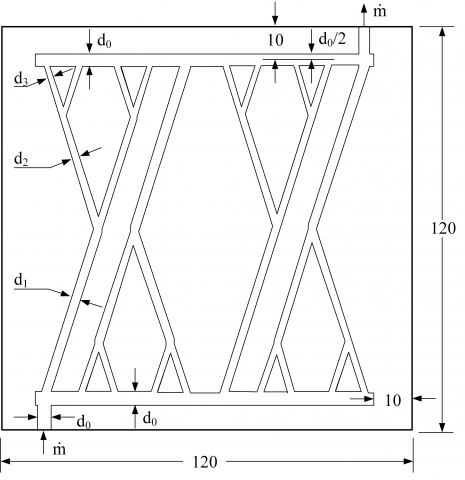Figure 5. Tree-shaped design configuration

The resistances to the fluid flow decrease with the tree-shaped channel configuration, i.e. mass flow rate increases in between 9% to 19% in tree-shaped configurations in comparison to the radial configurations while the pressure drop is fixed, which is in accord with the current literature . Figure 6a shows how the peak temperature varies relative to the pressure drop for two competing designs: radial and tree-shaped designs. The peak temperature is the greatest with radial design when the pressure drop is 10 Pa. However, the peak temperature of the radial design becomes smaller than tree-shaped design for a pressure drop range of 30 Pa to 290 Pa. Also, Figure 3 showed that semi-circular radial design provides smaller peak temperature in comparison with circular radial channel design when pressure drop is greater than 1500 Pa. Overall, Figure 3 and Figure 6a show that each design provides better cooling performance than another for a given pressure drop region. Therefore, we conclude that the design should be selected based on the given pressure drop value or the peak temperature value, which is in accord with the current literature.

Now consider the radial and tree-shaped designs with six outlet and inlet ports in order to uncover the effect of number of channels on the peak temperature. In radial design, there are six parallel channels which are connected to one distributing and one collecting channel of diameter d0. The tree-shaped design is also connected to the distributing and collecting channels of diameter d0 from the inlet and outlet ports. The channels of diameter d1 bifurcates into channels of diameter d2 at the junctions, i.e., the channels of diameter d3 is absent in Figure 5. Similarly, the volume fraction of the fluid domain and solid domain is fixed, however, it is φ=0.05 not φ=0.065. The volume fraction was decreased in order to have smaller channel diameter size than the thickness of the plate, i.e., as the number of the vascular channels decrease, the channel diameters increase because of the fixed volume constraint. Figure 6b shows how the peak temperature is affected when the number of vascular channel inlets and volume fraction are decreased from ten to six and from 0.065 to 0.05, respectively. Similar to Figure 6a, the peak temperature is smaller with tree-shaped design for a pressure drop of 10 Pa, and the peak temperature is smaller with radial design when pressure drop varies in between 30 Pa to 290 Pa. However, the effect of design becomes smaller with 6 channels and φ=0.05 as can be seen from the comparison of Figures 6a and 6b.

## 6.pngFigure 6. Peak temperature relative to the pressure drop for tree-shaped and radial design for (a) 10 daughter channels with φ=0.065, (b) 6 daughter channels and with φ=0.05

6. Hybrid Design

The radial and tree-shaped designs are superior to each other with the consideration of minimizing thermal and fluid flow resistances, respectively. Therefore, a hybrid of radial and tree-shaped designs is considered in order to minimize both fluid and heat flow resistances. Figure 7 illustrates the suggested hybrid design. Because the fluid flow resistances are smaller in tree-shaped designs, three additional radial channels of diameter d2 is added. These added branches decrease the thermal resistances in between cooling channels to decrease peak temperature. Because the volume fraction is a constraint, adding new branches decrease the diameters of the vascular channels. Even though, this decrease in the diameter increases the flow resistances, we are in search for a design that performs as good as radial design for cooling with the smallest pressure drop value.

Figure 8 shows how the peak temperature varies relative to three competing designs: tree-shaped, radial and hybrid designs for same volume fraction. Tree-shaped design provides the smallest peak temperature at the pressure drop of 10 Pa, however, its value only 0.5% lower than the peak temperature of the hybrid design. Therefore, we can conclude hybrid designs performs almost as good as tree-shaped design when the fluid resistances govern the peak temperature. Hybrid designs provide the smallest peak temperature when the pressure drop varies in between 30 Pa to 290 Pa. The difference between the peak temperature of the hybrid design and radial design decrease from 0.7% to 0.2% for 30 Pa and 290 Pa, respectively. This shows that as pressure drop increases, both radial and hybrid designs provides approximately the same temperature. Overall, Figure 8 shows that the peak temperature is the smallest or very close to the smallest with hybrid design for the entire pressure drop region for the given constraints and conditions.

## 7.png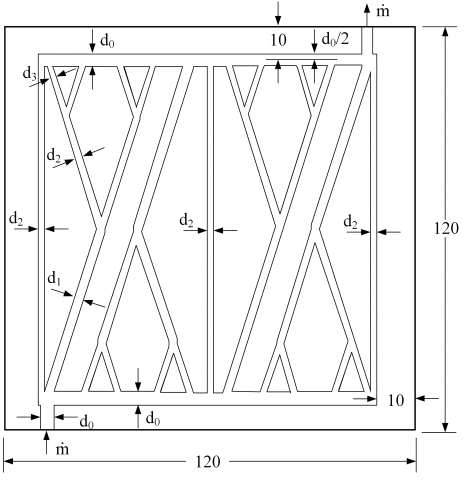Figure 7. Hybrid design configuration

## 8.pngFigure 8. Peak temperature relative to the pressure drop for three competing designs: tree-shaped, radial and hybrid

Figure 9 shows the temperature distribution on the top surface of the heated plate for competing designs of radial (circular), tree-shaped and hybrid designs for 30 Pa and 290 Pa. Figures 9a,c and e show that the farthest locations to the inlet port reach to the peak temperature. In addition, because the flow rate of the coolant is low, the coolant fluid temperature rises to the undesired levels. Moreover, Figure 9c shows that the hybrid design also promises the most uniform temperature distribution for 30 Pa. Figure 9b, d and f show the temperature distribution of the three competing designs for 290 Pa. Figure 9b shows that the radial configuration promises to provide uniform temperature distribution with small disturbances at the farthest channels from the inlet ports. However, Figure 9d shows that the temperature distribution is not uniform with tree-shaped channel configurations, i.e., the temperature is the smallest near the channel walls and it is the greatest in between and farther from the cooling channels. Therefore, even the fluid resistances are smaller in the tree-shaped design than in radial design, the conductive resistances in between the channels govern the temperature distribution. Figure 9f shows that the conductive thermal resistances can be decreased in between tree-shaped channels with embedded radial channels. Figure 9f also illustrates that both the peak temperature is minimum and the temperature distribution is the most uniform with hybrid designs for the given constraints and assumptions.

## 9.pngFigure 9. Temperature distribution of radial design for (a) 30 Pa, (b) 290 Pa, tree-shaped design for (c) 30 Pa, (d) 290 Pa, and hybrid design for (e) 30 Pa, (f) 290 Pa

7. Conclusions

Here, we showed that a plate with applied heating load on one of its surfaces can be kept under a desired temperature level with embedded cooling channels. The effect of the cross-section of the vascular channels on the cooling performance is uncovered for radial channel configuration. Circular channels provides greater cooling performance when the pressure drop is smaller. The semi-circular radial channels provides slightly better cooling performance (0.1% lower peak temperature) than circular channels as the pressure drop is in the order of 3000 Pa. The effect of volume fraction and number of channels on cooling performance are also documented, i.e., peak temperature decreases as φ increases, and decreasing the number of channels from 10 to 6 when φ=0.05 decreases peak temperature.

Then, the effect of changing the design from the radial configuration to tree-shaped configuration is uncovered with fixed volume fraction. Tree-shaped configurations provide smaller flow resistances than the radial configurations. Therefore, peak temperature is minimum with tree-shaped configurations when the pressure drop is small (i.e., 10 Pa in Fig. 6). However, conductive thermal resistances is a lot bigger in tree-shaped configurations than in radial configurations. Therefore, as the pressure drop increases, radial configurations provide better cooling performance. All these results lead us to suggest a hybrid design of radial and tree-shaped configurations. This hybrid design is created by placing radial channels in between tree-shaped channel configuration. We found that this hybrid design provides the smallest peak temperature (or very close to the smallest peak temperature) for the entire pressure drop region. We have also found that constraints and assumptions change the best performing design. Therefore, the design should be selected based on the constraints and limitations. This result is in accord with the constructal theory, i.e., there is no optimal design but the best performing design for a given time (conditions and constraints).

Acknowledgment

This research was funded by the scientific and technological research council of Turkey (TUBITAK) under grant number 114M592.

References

1. Shafahi, M., Bianco, V., Vafai, K., Manca, O., “Thermal performance of flat-shaped heat pipes using nanofluids,” International Journal of Heat and Mass Transfer, 53, 1438-1445, 2010.     DOI: 10.1016/j.ijheatmasstransfer.2009.12.007.

2. Li, Y., Xie, H.Q., Yu, W., Li, J., “Liquid cooling of tractive lithium ion batteries pack with nanofluids coolant,” Journal of Nanoscience and Nanotechnology, 15 (4), 3206-3211, 2015. DOI: 10.1166/jnn.2015.9672.

3. Alshaer, W.G., Nada, S.A., Rady, M.A., Del Barrio, E. P., Sommier, A., “Thermal management of electronic devices using carbon foam and PCM/nano-composite”, International Journal of Thermal Sciences, 89, 79-86, 2015. DOI: 10.1016/j.ijthermalsci.2014.10.012.

4. Ma, T., Yang, H.X., Zhang, Y.P., Lu, L., Wang, X., “Using phase change materials in photovoltaic systems for thermal regulation and electrical efficiency improvement: A review and outlook,” Renewable and Sustainable Energy Reviews, 43, 1273-1284, 2015. DOI: 10.1016/j.rser.2014.12.003.

5. Cetkin, E., Lorente, S., Bejan, A., “Vascularization for cooling a plate heated by a randomly moving source,” Journal of Applied Physics, 112 (8), 084906, 2012. DOI: 10.1063/1.4759290.

6. Cetkin, E., Lorente, S., Bejan, A., “Vascularization for cooling and mechanical strength,” International Journal of Heat and Mass Transfer, 54 (13-14), 2774-2781, 2011. DOI: 10.1016/j.ijheatmasstransfer.2011.02.061.

7. Wang, K.M., Lorente, S., Bejan, A., “Vascular materials cooled with grids and radial channels,” International Journal of Heat and Mass Transfer, 52 (5-6), 1230-1239, 2009. DOI: 10.1016/j.ijheatmasstransfer.2008.08.027.

8. Cho, K.H., et al., “Fluid flow and heat transfer in vascularized cooling plates,” International Journal of Heat and Mass Transfer, 53 (19-20), 3607-3614, 2010. DOI: 10.1016/j.ijheatmasstransfer.2010.03.027.

9. Rocha, L.A.O., Lorente, S., Bejan, A., “Tree-shaped vascular wall designs for localized intense cooling,” International Journal of Heat and Mass Transfer, 52 (19-20), 4535-4544, 2009.  DOI: 10.1016/j.ijheatmasstransfer.2009.03.003.

10. Cetkin, E., Lorente, S., Bejan, A., “Hybrid grid and tree structures for cooling and mechanical Strength,” Journal of Applied Physics, 110 (6), 064910, 2011. DOI: 10.1063/1.3626062.

11. Cho, K.H., Lee, J., Ahn, H.S., Bejan, A., Kim, M.H., “Fluid flow and heat transfer in vascularized cooling plates,” International Journal of Heat and Mass Transfer, 53, 3607-3614, 2010. DOI: 10.1016/j.ijheatmasstransfer.2010.03.027.

12. Cho, K.H., Chang, W.P., Kim, M.H., “A numerical and experimental study to evaluate performance of vascularized cooling plates,” International Journal of Heat and Fluid Flow, 32, 1186-1198, 2011. DOI: 10.1016/j.ijheatfluidflow.2011.09.006.

13. White, S. R., Sottos, N.R., Geubelle, P.H., Moore, J.S., Kessler, M.R., Sriram, S.R., Brown, E.N., “Viswanathan, S., Autonomic healing of polymer composites,” Nature, 409, 794-797, 2001. DOI: 10.1038/35057232.

14. Hamilton, A.R., Sottos, N.R., White, S.R., “Self-healing of internal damage in synthetic vascular materials,” Adv Mater, 22 (45), 5159-63, 2010. DOI: 10.1002/adma.201002561.

15. Bejan, A., Lorente, S., Wang, K.M., “Networks of channels for self-healing composite materials,” Journal of Applied Physics, 100 (3), 033528, 2006. DOI: 10.1063/1.2218768.

16. Wang, K.M., Lorente, S., Bejan, A., “Vascular structures for volumetric cooling and mechanical Strength,” Journal of Applied Physics, 107 (4), 044901, 2010. DOI: 10.1063/1.3294697.

17. Rocha, L.A.O., Lorente, S., Bejan, A., “Vascular design for reducing hot spots and stresses,” Journal of Applied Physics, 115 (17), 174904, 2014. DOI: 10.1063/1.4874220.

18. Cetkin, E., Lorente, S., Bejan, A., “Vascularization for cooling and reduced thermal stresses,” International Journal of Heat and Mass Transfer, 80, 858-864, 2015. DOI: 10.1016/j.ijheatmasstransfer.2014.09.027.

19. See www.comsol.com for information about COMSOL Multiphysics.

20. Bejan, A. and Lorente, S., Design with Constructal Theory, John Wiley & Sons, 2008. DOI: 10.1002/9780470432709.